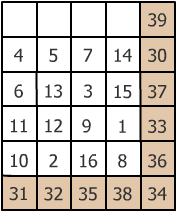#### You may also like### Consecutive Numbers

An investigation involving adding and subtracting sets of consecutive numbers. Lots to find out, lots to explore.### Calendar Capers

Choose any three by three square of dates on a calendar page...### Days and Dates

Investigate how you can work out what day of the week your birthday will be on next year, and the year after...

# Anti-magic Square

##### Age 11 to 14 ShortChallenge Level

The totals of the top row and completed main diagonal are 30 and 39 respectively and therefore the 10 consecutive numbers in question must be 30 to 39 inclusive.

The number in the bottom right hand corner must be 1, 2, 8, 15 or 16. Taking 1 and 2 the completed diagonal would add up to less than 30 whilst 15 and 16 produces a number greater than 39. Hence 8 must go in the bottom right hand corner. It now follows that the last digit in the second row must be 15 since if 15 is placed in any one of the other three vacant squares, we get a total of 45 in the second column, 34 in the third column (same as the diagonal) and 47 in the third row (too big). We are now left with 1, 2 and 16. End third row must be 1 to make the column less than 39 (38) and the row total 33. This leaves 2 and 16 making the forth row total. 16 cannot go in column 2 as this makes a total greater than 39.This problem is taken from the UKMT Mathematical Challenges.
You can find more short problems, arranged by curriculum topic, in our short problems collection.